# subtracting Fractions Problems

#### Number of problems found: 151

• When 5When 5 is subtracted from both my numerator and denominator I become 3/4. What fraction i am?
• A rectangle 7A rectangle that has been split into 24 parts, set in 4 rows of 6 parts each. The parts in the top 3 rows have been shaded blue. The parts in the left 5 columns have been shaded purple. The first 5 parts in the top 3 rows are striped to show they are shad
• ShopperEva spent 1/4 in one store and 1/3 in another. What fraction is left?
• Simplify expression with mixedWhen (7¹/3 + 2¾)÷(2 + 2¼ × 1¹/3) is simplified the result is?
• Evaluate 17Evaluate 2x+6y when x=- 4/5 and y=1/3. Write your answer as a fraction or mixed number in simplest form.
• Evaluate fractionsThe difference  of 5 1/2 and 2/3 is added to the product of 5/6 and 1/2
• Fraction expressionWhich expression is equivalent to : minus 9 minus left parenthesis minus 4 start fraction 1 divided by 3 end fraction right parenthesis
• DuringDuring a week, a student's spends 1/3 of her time in bed, 5/24 of her time in lesson and 1/8 of her time doing homeworks. What fraction of her time is left for doing other things?
• A farmer 3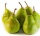A farmer sells 9.3 kg of pears and apples at the farmers market.3/4 of this weight is pears, and the rest is apples. How many kilograms of apples did she sell at the farmers market.
• Evaluate - lowest termsEvaluate: 16/25 - 11/25 (Express answer as a fraction reduced to lowest terms. )
• Human 3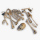Human bones are 3/10 living tissues 9/20 minerals, and the rest water. What fraction of human bone is water?
• Simplify 7Simplify. 7-1/3÷ 3-2/3 of 2+ 4-1/2÷ 2-1/4+ 1/2 solution and by step by step
• Claire and bookAfter Claire has read the first 5/8 of the book, there are 120 pages left to read. What is the total number of pages of the book?
• In bucket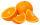In bucket of 87 oranges 1/3 are spoiled ones and the remaining are eaten by the member of a family. How many oranges are eaten by the member of family?
• Players - baseball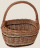There are 20 players on each of two baseball teams. If 2/5 of the players on team 1 miss practice and 1/4 of the players on team two miss practice, how many more players from team 1 missed practice then team 2?
• Equation with mixed 2A number, X, is subtracted from 8 1/4. The result is 12 3/5. What is the value of X?
• Complicated sum minus productWhat must be subtracted from the sum of 3/8 and 5/16 to get difference equal to the product of 5/8 and 3/16?Mike buys flowers to plant around his trees. 3/8 of the flowers are red. 1/3 of the flowers are pink. The rest of the flowers are white. Find the fraction of flowers that are white.
• Of the 2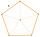Of the shapes,1/6 are triangles and 5/12 are pentagons. What fraction of the shapes are either triangles or pentagons?
• The dough 2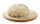A baker made 8 pounds of dough. He used 5/9 of the dough to make sandwich rolls. How much of the dough is left over?

Do you have an exciting math question or word problem that you can't solve? Ask a question or post a math problem, and we can try to solve it.

We will send a solution to your e-mail address. Solved examples are also published here. Please enter the e-mail correctly and check whether you don't have a full mailbox.

Please do not submit problems from current active competitions such as Mathematical Olympiad, correspondence seminars etc...

Need help to calculate sum, simplify or multiply fractions? Try our fraction calculator. Fraction Word Problems. Subtracting Problems.